Next: 4.2 DCIV Method Up: 4. Characterization of Interfaces Previous: 4. Characterization of Interfaces

Subsections

# 4.1 Charge Pumping Method

The charge pumping method has shown to be a very reliable and also precise method allowing the in-depth analysis of the interface, directly in the MOSFET device. Additionally it only requires basic equipment and is relatively easy to set up.

The effect has been first reported by Brugler and Jespers in 1969 . They reported a net DC substrate current when applying periodic pulses to the gate of a MOS transistor, while keeping source and drain grounded. The current was found to be proportional to the gate area and the frequency of the applied gate pulses. It was flowing in the opposite direction of the leakage current of the source and drain to substrate diodes. They showed that the current originates from recombination of minority and majority carriers at traps at the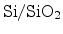interface. Therefore, the method can be used for measuring the interface trap density in MOSFETs for the evaluation of MOSFET degradation. The major breakthrough for the charge pumping method was the thorough investigation and correct explanation of the method, applied directly to MOSFET structures by Groeseneken et al. in 1984 .

## 4.1.1 Experimental Setup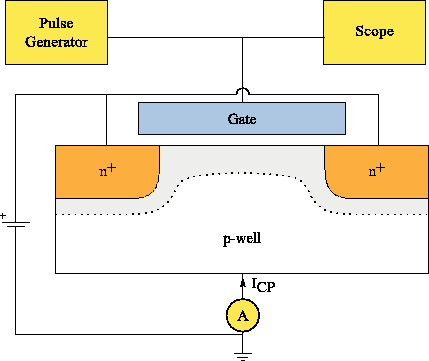The basic experimental setup for the charge pumping method can be seen in Figure 4.1 for an n-channel MOSFET. The source and drain to substrate diodes are reverse biased. The gate is pulsed between accumulation and inversion conditions while the charge pumping current is measured at the substrate. This current flows in the opposite direction of the source and drain to substrate diode leakage currents.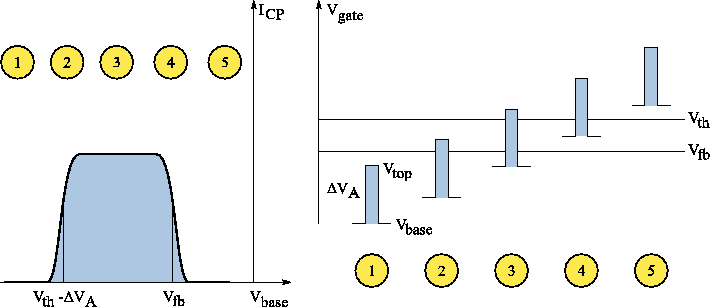In the accumulation phase majority carriers, holes in case of an n-channel MOSFET, flood the channel area and some of them become trapped in interface traps. When the gate pulse drives the transistor into inversion, the majority carriers leave the channel and move back to the substrate. Some trapped carriers with energies close to the valence band can be de-trapped through thermal emission before the channel becomes flooded by electrons and also move back to the substrate. The rest of the trapped holes recombines with channel electrons and leads to a net current. The same process occurs when the transistor is driven from inversion back to accumulation, with opposite carrier types.

The base level of the gate voltage pulse is swept to drive the MOSFET from accumulation to inversion. When the amplitude of the pulse is larger than the difference of threshold voltage and flat-band voltage,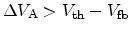, then five different regimes are observed as sketched in Figure 4.2. Regime 3, where the largest amount of traps in the band-gap is scanned, is the most important one. It can be described by a current model (Section 4.1.2) to calculate the interface trap density. The base level sweep charge pumping method was first proposed by Elliot  and the different regimes are governed by the following mechanisms:

#### 4.1.1.0.1 Regime 1:

The whole pulse is below the flat-band voltage and the substrate is in permanent accumulation. The interface traps are permanently filled with holes and therefore no recombination current is measured.

#### 4.1.1.0.2 Regime 2:

The top of the pulse reaches the region between the flat-band and the threshold voltages. In this phase the interface is moved from accumulation into strong depletion up to weak inversion. Here, the charge pumping current increases and the base voltage is around threshold voltage minus the pulse height. It could be assumed that the shape of the rising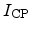in this regime is determined by the recombination process in weak inversion. It has been shown, though, that other mechanisms may have an important influence. These can be surface potential fluctuations because of spatially non-uniformly distributed oxide charges [42,43], acceptor and donor traps , or variations in the proximity of the source and drain regions. Also the modulation of the effective gate area by the gate voltage might influence the rising charge pumping current.

#### 4.1.1.0.3 Regime 3:

The base level voltage is below the flat-band voltage, and the top level of the pulse is above the threshold voltage,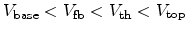. In this regime the charge pumping pulse sweeps the substrate in the channel area from accumulation to complete inversion. At each time the transistor is pulsed from accumulation to inversion or back. The fast interface traps are filled with holes, or electrons, respectively, which then recombine with the opposite carrier type leading to a net current measurable as. In this regime the current has the highest magnitude.

#### 4.1.1.0.4 Regime 4:

The base level is between the flat-band and threshold voltages. The transistor only reaches weak accumulation, the interface traps are mainly negatively charged and are no longer flooded with holes, thus recombination is reduced and the charge pumping current goes down. The same surface potential fluctuation and gate area modulation effects as in Regime 2 can influence the characteristic of.

#### 4.1.1.0.5 Regime 5:

The transistor is completely in inversion during the whole pulse. The traps are filled with electrons and no holes reach the channel at any time. The measured substrate current only consists of the source and drain leakage currents.

## 4.1.2 Charge Pumping Current Model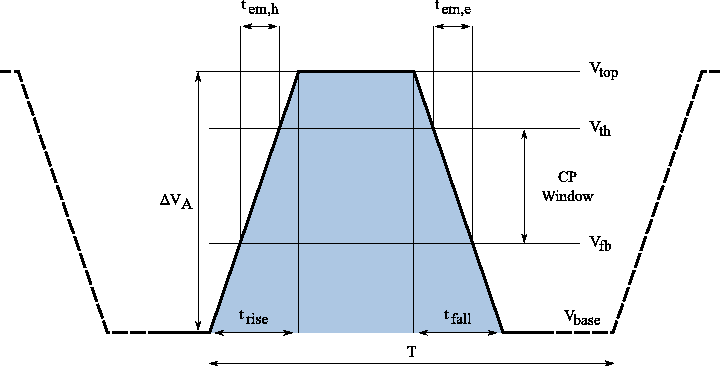The first comprehensive model for the charge pumping current was proposed by Groeseneken et al. in 1984 . This model has been developed to capture the maximum charge pumping current which is obtained in Regime 3. Here the gate voltage pulse sweeps from below the flat-band voltage to above the threshold voltage. Therefore the substrate is driven from accumulation to inversion and back.

Only the fast interface traps situated between the two energy levels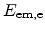and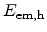in the band-gap of the semiconductor can contribute to the charge pumping current,(4.1)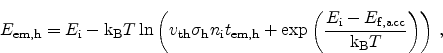(4.2)

where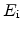is the intrinsic energy,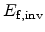and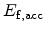are the Fermi energies in inversion and accumulation,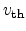is the thermal velocity,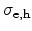the capture cross sections of the traps, and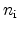is the intrinsic carrier concentration. Traps outside this band cannot contribute to the current as the trapped charge becomes de-trapped instantly through thermal emission when the Fermi-level moves beyond the trap level, for trapped electrons, or above, for trapped holes.

The emission times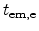and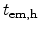for electrons and holes can be calculated from the fall and rise times,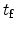and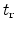, as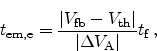(4.3)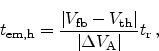(4.4)

and are illustrated in Figure 4.3. These are the times available for the emission of carriers from the fast traps.

The net CP current measured at the substrate can be obtained as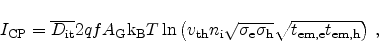(4.5)

whereis the frequency and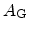the gate area.

The charge pumping current is directly related to the mean interface trap density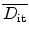in the channel, the size of theinterface channel area, the frequency, and the pulse shape characterized by its rise and fall times. This makes the charge pumping method a perfect tool for the characterization of interface degradation.

## 4.1.3 Numerical Simulation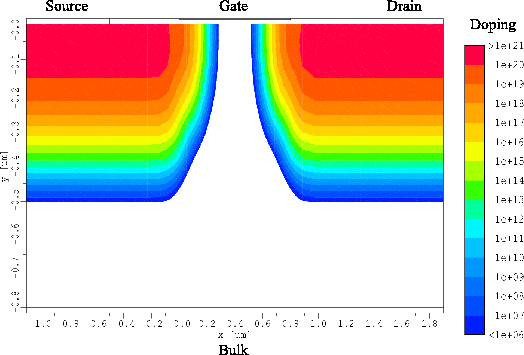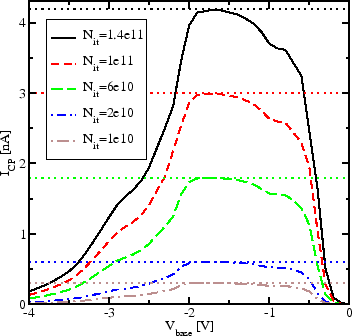The device simulator Minimos-NT  is used for numerical analysis of the charge pumping effect. For each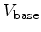of interest a transient simulation of the gate pulse is performed. The resulting currents can then be plotted versus the base voltage to obtain the typical charge pumping currentversusplot.

The device under test was a conventional n-channel MOSFET structure (Figure 4.4). The gate length, measured from source-substrate to substrata-drain junctions is 0.6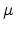m, the device width 100m, and the gate oxide thickness is 12nm.

### 4.1.3.1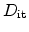Variation

The first simulation gives a comparison of the analytical current model (4.5) to numerical simulations using Minimos-NT. Here, the interface trap density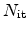has been varied from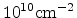up to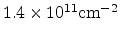, the pulse height is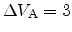V, and the rise and fall times are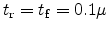s. Figure 4.5 gives the resulting CP curves and also the analytic approximation using (4.5). The agreement is excellent with the peak values of Regime 3.

### 4.1.3.2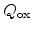Variation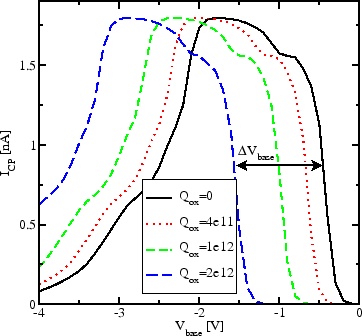The charge pumping method is very well suited for the quantitative investigation of fixed oxide charges. Figure 4.6 shows the charge pumping currents of a MOSFET device with constant density of interface traps but varying amount of fixed charges at theinterface. The oxide chargesare assumed to be located directly at the interface. The resultingin the numerical simulations for an interface charge density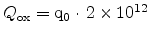cmis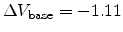V. This result is in excellent agreement with the analytical equation (2.31) presented in Section 2.2.1 and using the approximation for the plate capacitor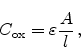(4.6)

where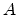is the area and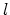is the dielectric thickness, predicting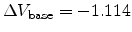V.

### 4.1.3.3 Pulse Amplitude Variation

Figure 4.7: Variation of the gate pulse height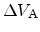. The interface trap density is constant at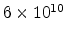cm.strongly increases until. The marginal increase at higheris due to the decreasing emission times.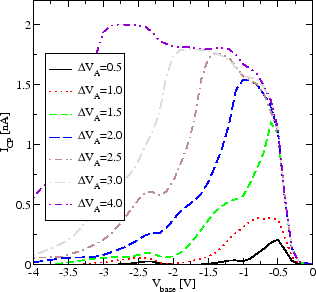Linear scale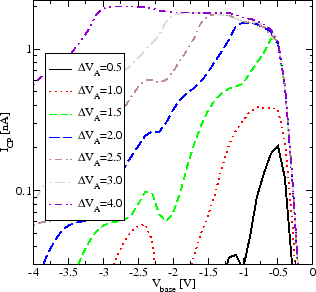Logarithmic scale
Experimenting with the pulse heightnicely illustrates the strongly increasing charge pumping current untilsurmounts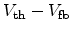(Figure 4.7).

It can be seen that for increasing gate amplitudes the saturation current still slightly increases for. This effect is because the transistor's depletion region is swept faster resulting in decreasing emission times for electrons and holes, (4.3) and (4.4). Therefore, the thermal emission is reduced and more traps can contribute to the charge pumping current.

### 4.1.3.4 Reverse Bias

Figure 4.8: Variation of the source/drain to substrate bias. The interface trap density is kept constant atcm. At high bias the source/drain to substrate diodes are turned off. At lower source and drain voltages the source/drain to substrate diodes are turned on and the diode currents dominates the charge pumping current (belowV).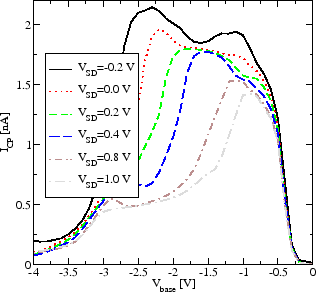Linear scale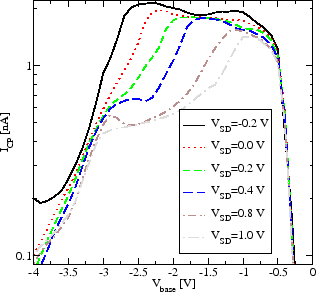Logarithmic scale
By increasing the reverse bias the charge pumping current is decreased, as shown in Figure 4.8. This reduction is due to two effects:
1. The body effect. It leads to an enlargement of the space charge region and therefore to an increase of the threshold voltage. This increased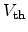in turn increases the emission times for electrons and holes, (4.3) and (4.4), and therefore to a reduction ofas found from (4.5).
2. Due to the increase of the space charge regions around source and drain during accumulation the effective channel gate area is reduced. Therefore, less interface traps can contribute to the charge pumping current.

For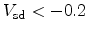V the charge pumping current is dominated by the source/drain to substrate diode current and cannot be used for the evaluation of interface traps.

### 4.1.3.5 Temperature Dependence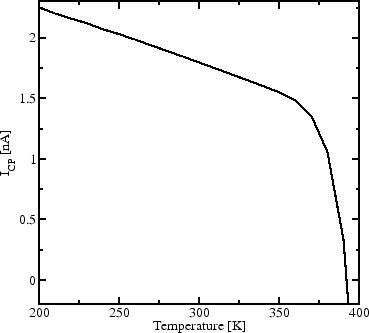As the thermal emission process is, as its name already suggests, strongly temperature dependent, so is the charge pumping current. At higher temperatures more carriers can be de-trapped before recombining with the opposite carrier type andis reduced (Figure 4.9).Next: 4.2 DCIV Method Up: 4. Characterization of Interfaces Previous: 4. Characterization of Interfaces

R. Entner: Modeling and Simulation of Negative Bias Temperature Instability